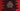# Java example program to left shift an array### Java program to left shift an array :

In this tutorial, we will learn how to left shift an array in Java . Left shifting by one means the array elements will be moved by one position to left and the leftmost element will be moved to end.

For example, if the array is [1,2,3,4], left shifting will make it [2,3,4,1]. In this tutorial, we will learn how to do left shifting of an array in Java. Our program will use one predefined number array but it will work with any other arrays. We will use one different function to do the shifting.

### Java Example Program :

``````import java.util.Arrays;

public class Main {

public static int[] shiftLeft(int[] array) {
//4
int startNumber = array;
//5
System.arraycopy(array, 1, array, 0, array.length - 1);
//6
array[array.length - 1] = startNumber;
//7
return array;
}

public static void main(String[] args) {
//1
int[] numbers = {1, 2, 3, 4, 5, 6, 7, 8, 9, 10};

//2
System.out.println("You have entered : " + Arrays.toString(numbers));

//3
int[] finalArray = shiftLeft(numbers);

//8
System.out.println("After left shift : " + Arrays.toString(finalArray));

}

}``````

### Output :

``````You have entered : [1, 2, 3, 4, 5, 6, 7, 8, 9, 10]
After left shift : [2, 3, 4, 5, 6, 7, 8, 9, 10, 1]``````

### Explanation :

The commented numbers in the above program denote the step numbers below :

1. numbers is an integer array with 10 integer values.
2. Print the array to the user.
3. Call the shiftLeft method. It takes one integer array as the argument and returns the new array. Pass numbers as the argument.
4. Store the first element of the array in startNumber variable.
5. Use the method arraycopy to copy all elements from second to the end index to the same array from start to end-1 index.
6. Set the value of startNumber to the end of the array.
7. Return the array.
8. Finally, print the new array.